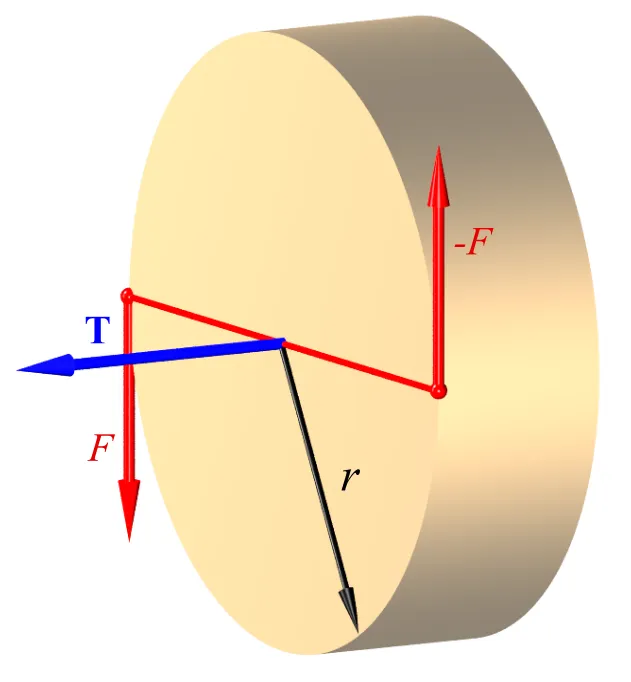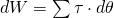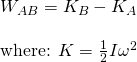# Physics - Classical Mechanics - Work and Power in Angular Motion[Image 1]

## Introduction

Hey it's a me again @drifter1! Today we continue with Physics and more specifically the branch "Classical Mechanics" to get into Work and Power in Angular Moton. So, without further ado, let's get straight into it!

## Work in Angular Motion

In angular motion we define the change in position as the change of the angle θ. With that in mind think about a rigid body that rotates through an angle from some point A to point B, while a force F is applied to a point P. As we described in a previous article, the position of this point is defined by the vector r. To make the calculation procedure simpler, let's suppose that the rigid body is constrained to rotate about a fixed axis O. That way vector r moves in a circle of radius r. The arc length s of the circle if equal to s = θ·r. Because vector r moves in a circle, and by also defining the change in angle as a vector θ we can end up with the following cross product that will give us the change in arc length as a vector:From the small change in angle and because dr = 0, from the assumption of having the rigid body fixed on O, we get:From the definition of Work in integral form we obtain:So, the total work done on a rigid body is equal to the sum of torques integrated over the angle which the body rotates. A more descriptive way of writing the formula is:where someone can clearly see the change from some angle A to B

If the complete body rotates through the same angle the so called incremental work is equal to the sum of torque times the common incremental angle dθ:## Work-Energy Theorem

Similarly to Translational motion, the Work-Energy Theorem also works for Angular motion. Thinking about a rigid body rotating around a fixed axis we have:For Rolling motion (translational + angular) the Kinetic Energy for A and B will be equal to the sum of each kinetic energy, as we described in the previous article:## Power in Angular Motion

As you might remember, Power is equal to rate of doing Work. So, it's the change of work over time. Thinking about a constant net torque and the simple equation of Work that we defined previously (W = τθ), the power of angular motion is given by the equation:Examples around all the equations that we covered today and in the most articles, will be done in a separate article, when we are finished with angular motion!

## RESOURCES:

### References

1. https://phys.libretexts.org/Bookshelves/University_Physics/Book%3A_University_Physics_(OpenStax)/Map%3A_University_Physics_I_-_Mechanics%2C_Sound%2C_Oscillations%2C_and_Waves_(OpenStax)/10%3A_Fixed-Axis_Rotation__Introduction/10.8%3A_Work_and_Power_for_Rotational_Motion
2. https://opentextbc.ca/physicstestbook2/chapter/rotational-kinetic-energy-work-and-energy-revisited/
3. http://farside.ph.utexas.edu/teaching/301/lectures/node105.html

### Images

1.## Final words | Next up

This is actually it for today's post! Next time we will get into Angular Momentum...and the "famous" Conservation of Angular Momentum!

See ya!Keep on drifting!

H2
H3
H4
3 columns
2 columns
1 column